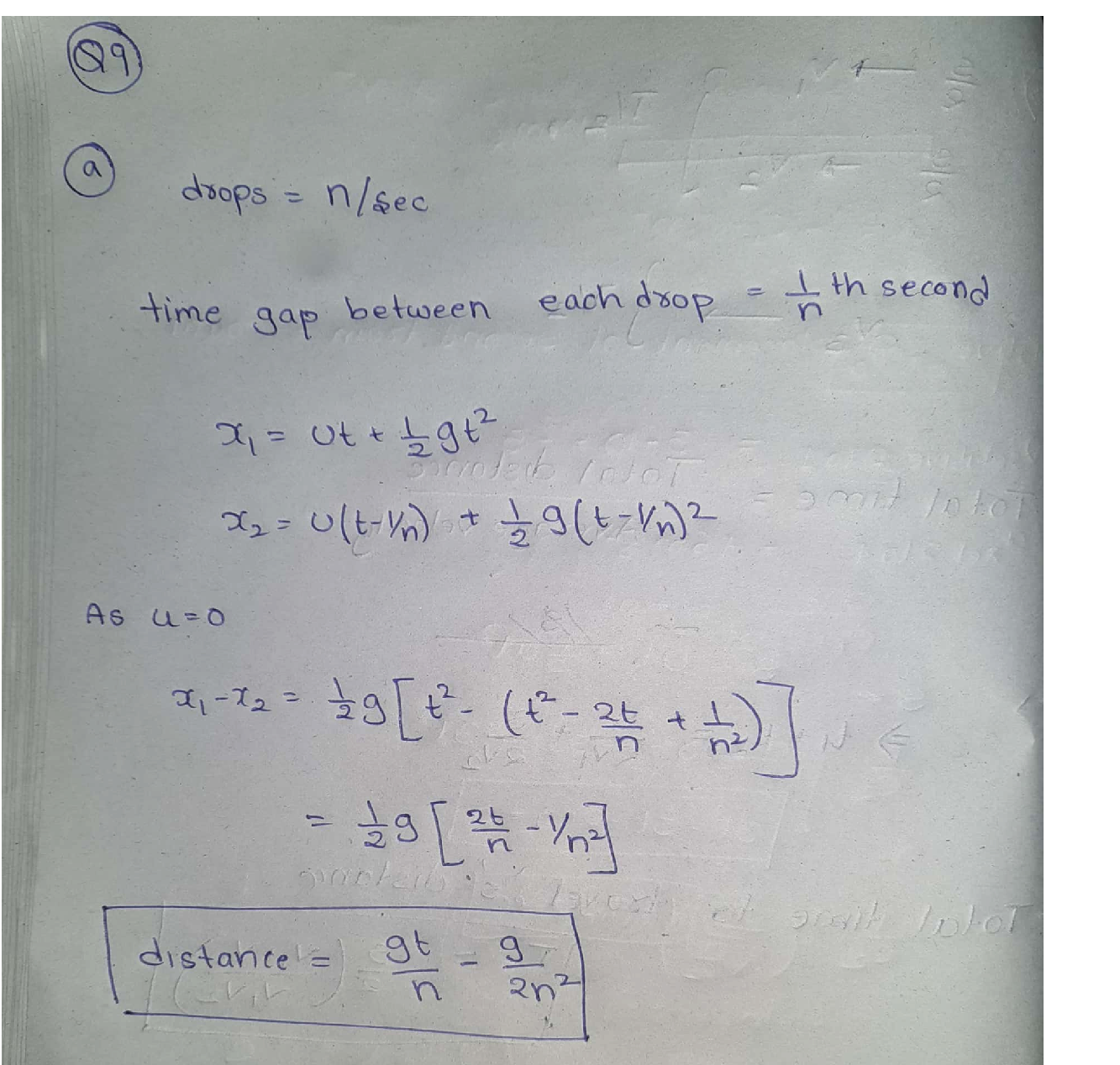Question

Modern Physics

Problem 9: The first man to study and partially understand motion under constant acceleration was Leonardo da Vinci (1452 – 1519), almost two centuries before calculus was invented. He describes, in his famous notebooks, the apparatus shown consisting of two vertical boards, hinged together on one side and covered with blotting paper on the inside faces. A leaking water faucet lets drops fall between the boards at equal intervals of time. Using a string mechanism, the boards can be quickly clapped together and the position of the drops on the blotting paper can be inspected. Leonardo da Vinci observed that the distance between consecutive drops increased in a “continuous arithmetic progression."!

(a) If the water droplets drip from the faucet at a uniform rate of n drops per second, find the distance x between any two adjacent drops as a function of the time t that the trailing drop has been in motion. Neglect air resistance. Assume that the acceleration due to gravity, g, is constant.

(b) Suppose that the vertical boards are 3 ft long. The leaking faucet is at such a height, and therate of water droplets falling is so regulated, that when a drop is just coming out of the faucet,the next or second drop is just at the top of the boards, and the sixth drop is at the bottom ofthe board. Calculate the number of drops leaving the faucet each second. Also determine thedistances between the six drops of water.Verified### Question 52422Modern Physics

1A cruiser moves away from the planet Hoth at a speed of 0.5c. A frigate follows, catching up at a speed of 0.25c as viewed from the cruiser. With what speed does the frigate appear to be moving according to observers on the planet? You should use the relativistic velocity transformation, clearly defining all the parameters used.
u^{\prime}=\frac{u-v}{1-\frac{u v}{c^{2}}}

### Question 44658Modern Physics

Question 5: A spaceman goes out on a trip. First, he travels at 1/3 the speed of light in one direction. Then he comes back home at 2/3 of the speed of light. On Earth 9 years have passed,by how much has the spaceman aged? (Make sure you draw a space-time diagram).

### Question 44657Modern Physics

Question 4: The typical pole for pole vaulting is 17 feet long. How fast would a pole vaulter have to run (in the direction of the pole) in order for a spectator sitting in the stands to see it 15feet long?

### Question 44656Modern Physics

Question 3: If you weigh 150 lb in Chicago, how much do you weigh on top of mount Everest?(mount Everest is 8,848 m high).

### Question 44655Modern Physics

Question 2: As a result of an explosion a stationary object breaks into three pieces. One piece flies east, one west and one north.
Explain why this is impossible.
Now, there are only two pieces; one flies east the other west. One piece weighs three times as much as the other. What is the ratio of the kinetic energies of the two pieces?

### Question 44654Modern Physics

Question 1: The international Space Station (ISS) orbits the Earth once every 90 mins at an-altitude of 409 km. How high would it have to be in order to be to be in geosynchronous orbit?(make sure you show how you worked it out).

### Question 44179Modern Physics

Question 6: A "star" made of ideal gas radiates away 10% of its energy while remaining in hydrostatic balance. Assuming that there are no nuclear reactions
Does the radius of the "star" increases or decreases? By how much?
Does the (average) temperature of the "star" increases or decreases? By how much?

### Question 44178Modern Physics

5: There are two stars, one has twice the luminosity of the other and half the radius.
What is the ratio of their temperatures?
What is the ratio of their (apparent) brightness if the more luminous one is 100 times more distant than the other.

### Question 44177Modern Physics

Question 4: According to general relativity people who live in hi-rise buildings age at differentrates depending on their floor.
Is that true?
Which floor ages fastest?

### Question 44176Modern Physics

Question 3: Consider two time-like events P and Q. P is at the space-time origin P=(0,0); Q is at the space origin but one second later Q=(0,1).
Show that the order of events is preserved by all observers travelling at less than the speed of light.
Now events P and Q are space-like. P is still at the origin, but Q is simultaneous with P but one meter to the right 0=(1.0)
.Show that the order of events is not preserved by observers travelling at less than the speed of light.

### Submit query